﻿ Nonlinear Dynamics of a Controlled Cantilever Beam with Varying Orientation under Primary Resonance

### Nonlinear Dynamics of a Controlled Cantilever Beam with Varying Orientation under Primary Resonance

Usama H. Hegazy

American Journal of Mechanical Engineering

## Nonlinear Dynamics of a Controlled Cantilever Beam with Varying Orientation under Primary Resonance

Usama H. HegazyDepartment of Mathematics, Faculty of Science, Al-Azhar University, P.O. Box 1277, Gaza, Palestine

### Abstract

The problem of controlling the oscillations and chaotic behavior of a nonlinear cantilever beam with varying orientation under mixed excitations is tackled. Numerical integration of the second order nonlinear ordinary differential equation is performed with different control strategies to explore the chaotic dynamics of the first mode of the beam at the primary resonance case. The method of multiple scales perturbation technique is applied to obtain approximate solution and the stability of the response is studied. The effects of the various parameters are investigated by numerical simulations.

• Usama H. Hegazy. Nonlinear Dynamics of a Controlled Cantilever Beam with Varying Orientation under Primary Resonance. American Journal of Mechanical Engineering. Vol. 2, No. 7, 2014, pp 316-327. http://pubs.sciepub.com/ajme/2/7/31
• Hegazy, Usama H.. "Nonlinear Dynamics of a Controlled Cantilever Beam with Varying Orientation under Primary Resonance." American Journal of Mechanical Engineering 2.7 (2014): 316-327.
• Hegazy, U. H. (2014). Nonlinear Dynamics of a Controlled Cantilever Beam with Varying Orientation under Primary Resonance. American Journal of Mechanical Engineering, 2(7), 316-327.
• Hegazy, Usama H.. "Nonlinear Dynamics of a Controlled Cantilever Beam with Varying Orientation under Primary Resonance." American Journal of Mechanical Engineering 2, no. 7 (2014): 316-327.

 Import into BibTeX Import into EndNote Import into RefMan Import into RefWorks

12345
Prev Next

### 1. Introduction

A single degree of freedom flexible beam subjected to a vertical sinusoidal base excitation that has a mass-pendulum attached to its tip is investigated. It is found that the best performance of the pendulum is observed at the horizontal orientation of the system. Moreover, the energy is absorbed from the system by the absorber until a critical boundary is reached . The vibration control of one dimensional cantilever beam of varying orientation under external and parametric excitations is studied and analyzed. The cubic velocity feedback control is applied and the method of multiple scaled is utilized to construct the modulation equations of the amplitudes and phases. Numerical simulations are performed to investigate the effects of system parameters and the stability . The nonlinear vibration and saturation phenomenon are analyzed in the controlled hinged-hinged flexible beam. The performance of different control techniques are studied and found that the oscillations of the system can be controlled actively via negative velocity feedback. Effects of system parameters are also investigated . A control law based on quintic velocity feedback is proposed to reduce the vibrations of one dimensional cantilever beam under primary and parametric excitations. Numerical simulations are performed to verify analytical results obtained using the multiple scales method and to detect chaos and unbounded motions . The vibrations of a rotor-active magnetic bearing (AMB) system at primary resonance and the presence of one-to-one and one-to-two internal resonances are studied and suppressed applying saturation-based active controller. Optimum working conditions are obtained to help improve the design of the system . Positive position feedback active controller is applied to reduce the oscillations of a nonlinear system to external primary resonance excitation. The multiple scales method is utilized to obtain a first-order approximate solution, which is numerically verified . A nonlinear time delay saturation-based controlled is applied to suppress the vibrations of a beam when subjected to external excitation considering simultaneous primary and two-to-one internal resonance case. Bifurcation analysis is performed to determine the stability of the closed loop system and to study the effectiveness of the control law . The nonlinear behavior of a cantilever beam subjected to external and parametric excitations is studied. The cases of primary and subharmonic resonances are considered and the multiple scales method is used to obtain two first order ordinary differential equations. Effects of different parameters are investigated and the approximate solution is verified numerically . A two degree-of-freedom model of a nonlinear vibration absorber to external excitation at the simultaneous primary resonance and one-to-one internal resonance is investigated applying two control strategies for positive and negative delayed feedback. Analytical solutions are obtained using the method of multiple scales and are found to be in good agreement with numerical simulations . The method of multiple scales is utilized to obtain analytical solution for the nonlinear differential equations describing the motion of the controlled electromechanical system with time-varying stiffness. Negative linear, quadratic, and cubic velocity feedback controllers are applied to the system and investigated. It is found that the vibration of the seismograph model is best controlled via the negative velocity feedback . A nonlinear cantilever beam of varying orientation with time-delay subject to direct and parametric excitations is studied at the primary and parametric resonances. The first order approximation of the response is obtained using the multiple scales method, where the time delay is presented in the proportional feedback and the derivative feedback . The Melnikov method is used to determine the homoclinic and heteroclinic chaos in the micromechanical resonators. Numerical simulations including basin of attraction and bifurcation diagrams reveal the effect of parametric excitation on the system transition to chaos and confirm analytical predictions. Moreover, the time-varying stiffness is introduced to control the chaotic motion of the system . RCL circuits with variable capacitance is applied to control the oscillations in a hinged-hinged beam based on the variational principle. The controlled system is investigated by Numerical simulations deal with the optimization of the control law and analytically to find the condition of the control . A differential control method based on the mechanized mathematics-Wu elimination method is proposed to solve the chaotic solutions of high-dimensional nonlinear dynamic systems . The finite element method is used to investigate the nonlinear response of Timoshenko beam attached with tuned mass damper (TMD) traversed by a moving vehicle. The optimum values of the frequency and damping ratio of TMD are determined to minimize the maximum frequency response of the beam midspan . A dynamic beam structure system is controlled applying a feasible methodology based on time-delayed boundary control. Wavelets are employed to solve time-delayed linear lumped parameter system. The efficiency of the proposed control algorithm is investigated by numerical simulations . Multi-input delay-feedback controllers based on the damping produced by these controllers as a function of the gains and delay are proposed and analyzed to suppress free oscillations for the first mode of the cantilever beam. Optimal values of the controllers gains and delay are obtained. Numerical simulations using three-mode nonlinear beam are carried out to validate the single mode approximation . Longitudinal and transverse vibrations of an axially moving string system are reduced applying an active control scheme, which also regulates the transport velocity of the system to track a desired moving velocity profile. Three inputs are used in the control scheme, which are generated by the Lyapunov method. Numerical simulations are performed to illustrate the effectiveness of the proposed control scheme . Acceleration feedback (AF) controller with a sensor/moment pair actuator configuration is used to control actively clamped-clamped beam. The gain and damping ration are shown to affect significantly the stability and performance of the AF controller . The effect of an attached nonlinear energy sink on energy reduction of a cantilever beam subject to shock excitation is investigated. The role of nonlinear normal modes of both systems in determining the dynamics of energy pumping is studied to identify the required conditions of targeted energy transfer .

In this study, controlling chaotic dynamics and suppressing primary response of a nonlinear beam of varying orientation under direct and parametric excitations are studied and analyzed. The equation of motion of the beam is reduced by the multiple scales method to two first order nonlinear ordinary differential equations, governing the amplitude and the phase of the first mode motion. The performance of various control laws is analyzed by numerical simulations. Analytical solution under two best controllers is illustrated in both frequency-response curves for the primary resonant case. Numerical and theoretical results are compared and found to be in good agreement.

### 2. Mathematical Model

The governing equation of motion to be studied and solved has the from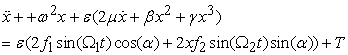(1)

where

ε is a small bookkeeping perturbation parameter, µ is the damping coefficient, ω is the natural frequency, f1 and f2 are the external and parametric forcing amplitudes, Ω1,2 are the forcing frequencies, β and γ are quadratic and cubic nonlinear terms, α is the orientation angle of the beam and T is a control input.Download asVeiw figureFigures index
Figure 1. The considered model of a cantilever beam

### 3. Time-history Solution and Control

In this section, the cantilever beam model (1) is numerically integrated using fourth order Runge-kutta algorithm to obtain nonresonant time-series solution and the system behavior at primary resonance condition. Then, different controllers will be applied and investigated at the resonant case. Figure 2 shows time histories and phase portraits of the considered model when the values for the system parameters are chosen as follows:

µ=0 .02, ω = 10.9, f1 = 1.5, f2 = 1.2, Ω1 = 10.4, Ω2 = 9.9, β = 10.0, γ = 2.03, α = 10o with initial condition x(0)=0.1 and zero initial velocity, unless otherwise specified.

3.1. Effect of Natural and Excitation Frequencies

When Ω = ω (primary resonance), the maximum steady-state amplitude has increased to about 445%, compared to the non-resonant case shown in Figure 2(a). This resonant condition is shown in Figure 2(b) and will be considered as basic case in our study.

3.2. Effect of Initial Conditions

It can be seen from Figure 2(d) that at the beginning, as the initial condition x(0) is varied from 1.0 to 3.0, the transient time is increased. But the steady-state amplitudes are the same for all initial conditions different values. This means that the system depends on initial conditions, which is a characteristic of a nonlinear system.Download asVeiw figureFigures index
Figure 2. Numerical solution of the cantilever beam: (a) Non-resonant time response solution, (b) Primary resonant solution, (c) Effect of initial conditions on system behavior at primary resonance
3.3. Effect of the Orientation Angle (α)

Figure 3(a) and Figure 3(b) show that small angles between 10o and 50o does not significantly affect the amplitude of the beam. When the orientation angle is increased to 80o, Figure 3(c), the amplitude decreases and the tuned behavior disappears. As α is increased further, the reduction in the amplitudes is noticed significantly and the system reaches the steady state as shown in Figure 3(d).Download asVeiw figureFigures index
Figure 3. Effect of the orientation angle under primary resonance
3.4. Effect of the Forcing Excitation Amplitudes
3.4.1. Direct Excitation f1

It can be shown from Figure 4, that the amplitude of the model increases as the excitation amplitude of the external force increases.

3.4.2. Parametric Excitation f2

The effect of the amplitude of the parametric excitation f2 is shown in Figure 5, which represent the time-series solution (t, x) and the phase plane (x, v) for the beam at primary resonance, where v denotes the velocity. Considering Figure 2(b) as basic case for comparison. It can be seen from Figure 5(a) that as the amplitude of parametric excitation f2 is increased to 100.0, a chaotic motion occurs. When f2 is increased to 200.0, a modulated chaotic behavior is noticed in Figure 5(b). The time history of the beam, Figure 5(c), shows a change from modulated chaotic response to a periodic response as f2 is increased further to 400.0. More increase lead to a severe chaotic motion as indicated in Figure 5(d).Download asVeiw figureFigures index
Figure 4. Effect of the external excitation under primary resonanceDownload asVeiw figureFigures index
Figure 5. Effect of the parametric excitation under primary resonance
3.5. Vibration Control

Different controllers have been applied to the cantilever beam model, which are the position feedback, the negative velocity feedback and the negative acceleration feedback controllers. The performance of several types of each kind of the three controllers is studied and analyzed at the primary resonance response of the system. Figure 6(a) is considered as basic case for comparison.

3.5.1. Position Feedback (PF) Laws,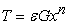(i) When n = 1, it is called the linear PF controller and it is aimed at modifying the natural frequency of the system. Figure 6(b) - Figure 6(d), show the performance of controller for different values of the gain G. It is clear that the effect of the controller becomes significant in reducing the amplitude of vibration for G > 5.0. Larger values for the gain may not be effective enough compared to the percentage of reduction in the amplitude.

(ii) When n = 2, the effect of the quadratic PF is shown in Figure 6(e) and Figure 6(f), which indicates that the reduction in the amplitude is noticeable when G takes very large values (G > 70.0).Download asVeiw figureFigures index
Figure 6. Performance of LPF controller, (a)-(d), and QPF controller, (e) and (f), for different values of the gain when the system subjected to primary resonance

(iii) When n = 3, the cubic PF controller is activated and affects the cubic nonlinearity coefficient in the beam system due to nonlinear curvature when G > 20.0 as indicated in Figure 7.

It is clear that increasing gain of all PF controllers is not only suppressing the amplitude of vibration but changes the oscillations of the beam to a strongly modulated motion as well.Download asVeiw figureFigures index
Figure 7. Performance of CPF controller for different values of the gain when the system subjected to primary resonance

3.5.2. Velocity Feedback (VF) Laws,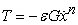(i) When n = 1, it is called the linear VF controller and it modifies the damping of the considered system. It can be seen from Figure 8 that the controller is very effective in suppressing the amplitude of the system for small values of the gain G and the motion is steady.Download asVeiw figureFigures index
Figure 8. Performance of LVF controller for different values of the gain when the system subjected to primary resonance

(ii) When n = 2, Figure 9 indicates that the quadratic VF controller may not be considered to have a good criterion among active controllers for the considered beam. In fact, the beam looks to be sensitive to increasing the gain, which resulted in affecting the shape of the motion.

(iii) When n = 3, a significant reduction of the system amplitude is observed in Figure 10 for very small values of the gain G as the cubic VF controller is added to the system. Also the behavior of the system is noticeably affected by the controller to reach the steady-state.Download asVeiw figureFigures index
Figure 9. Performance of QVF controller for different values of the gain when the system subjected to primary resonanceDownload asVeiw figureFigures index
Figure 10. Performance of CVF controller for different values of the gain when the system subjected to primary resonance

3.5.3. Acceleration Feedback (AF) Law,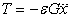It is clear that this control law modifies the acceleration of the system. The AF controller may act better for G < 0.2. More increase may lead to unstable system as shown in Figure 11.Download asVeiw figureFigures index
Figure 11. Performance of negative acceleration feedback controller for different values of the gain when the system subjected to primary resonanceDownload asVeiw figureFigures index
Figure 12. Effect of the gain for various control laws at primary resonance - numerical integration of the system: (x-axis: the gain, y-axis: x-amplitude)

3.5.4. Effect of the Gain Coefficient G

Figure 12 shows the effect of the amplitude of vibration with respect to the gain for the PF and VF control laws. The effectiveness of the different active control laws is evaluated to determine the one that leads to effective vibration suppression and chaotic motion control of the system. The effectiveness of the controller is represented by Ea = steady-state amplitude of the system before control / steady-state amplitude of the system after control, which is calculated at saturation beginning. Comparing the effectiveness of these controllers. It is noticed from Figure 12(a) and Figure12(b) that

Ea = 15.3, 3.32, and 2.68 and saturation begins at G greater than 30.0, 75.0, and 20.0 to control the system when applying LPF, QPF, and CPF controllers, respectively. This means that the LPF controller (T = Gx) is the best one among PF controllers, which has better performance when 1.0 < G < 5.0.

Ea = 31.0, 5.5, and 19.0 and saturation begins at G greater than 5.0, 2.0, and 5.0 to control the system when applying LVF, QVF, and CVF controllers, respectively. This means that the LVF controller (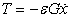) is the best one among VF controllers. Comparing the LVF and CVF controllers, one observed that for G < 1.0 the LVF and CVF laws have same effect, while the CVF controller is better than the LVF one when 1.0 < G < 4.0 as shown in Figure 12(b)(i). But Figure 12(b)(ii) indicates that as G increases beyond 4.0 the linear VF controller performes better.

Therefore, the best control method among all applied controllers is the LVF one.

### 4. Analytical Solution

In this section the approximate solution of the nonlinear system, Eq. (1), with the two effective controllers is analyzed, applying the method of multiple scales.

4.1. LPF Controller

Setting T = εGx in Eq. (1) and assuming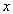is in the form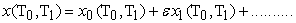(2)

where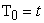is the fast time scale and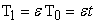is the slow time scale. The time derivatives are expressed as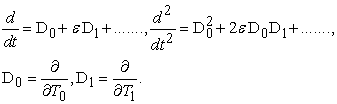Equating the coefficient of same powers of ε yields: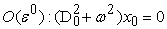(3)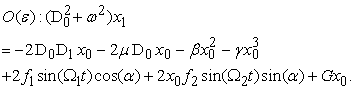(4)

The general solution of Eq. (3) is given by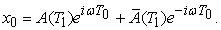(5)

Substituting Eq. (5) into Eq. (4) , gives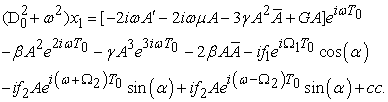(6)

The particular solution of Eq. (6) is given by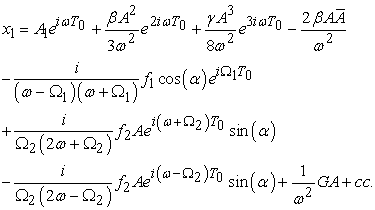(7)

The primary resonance, due to external force, will be considered and studied. It occurs when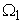is very close to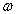, which is expressed as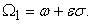(8)

From Eq. (6) the secular terms, which result in unbounded solution, are eliminated and the solvability condition yield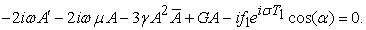(9)

Using the following polar form expression in Eq. (9)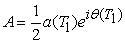(10)

where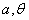are the steady-state amplitude and phase of the motion. Then Separating imaginary and real parts, and setting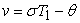we obtain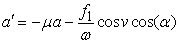(11)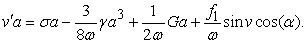(12)

The steady-state solutions correspond to constant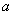,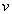that is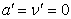Thus Eqs. (11) and (12) can be reduced to the following nonlinear algebraic equations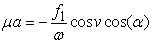(13)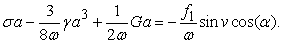(14)

Squaring Eqs. (13) and (14) then adding them, we obtain the following frequency response equation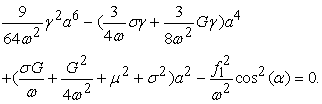(15)
4.2. LVF Controller

Setting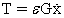in Eq. (1) and following the same procedure as in LPF controller considering the primary resonance case, one obtains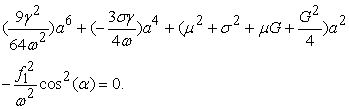(16)

Next, we study the problem of stability of linear (trivial) solutions for the two considered controllers.

4.3. Trivial Solution

To determine the stability of the trivial solutions, one investigates the primary resonant solution with LPF controller by introducing the following Cartesian form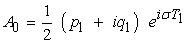(17)

into the linearized form of Eq. (9), that is into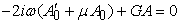(18)

where p1, q1 are real, then separating real and imaginary parts, gives the following eigen-value equation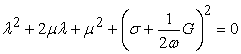(19)

which has the solution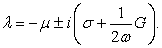(20)

In a similar manner, one can obtain the following eigenvalues for the stability of the trivial primary resonant solution with LVF control law as follows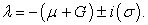(21)

Consequently, the linear solution is stable for all negative values of the real part of the obtained eigenvalues.

4.4. Analytical Results

In this section, the solution of the frequency response Eqs. (15) and (16) are obtained numerically. The stability of the steady-state solution is investigated using frequency response function and the numerical results are focused on the effect of different parameters. The results of solving Eqs. (15) and (16) are shown in Figure 13 and Figure 14 as the amplitude a against the detuning parameters σ, which are called frequency- response curves.

4.4.1. LPF Controller

Figure 13(a), which is considered a basic case to compare with, indicates that the system under L PF controller possesses a strong hardening nonlinearity effect. it can be seen from Figure 13(b) that as the damping coefficient µ increases the steady-state amplitude a decreases. The effect of varying the natural frequency ω is shown in Figure 13(c), where the region of multi-valudeness and stability between the two branches is decreased as ω increases. Figure 13(d) shows that the frequency response curves are bent to right when the nonlinear term γ is positive and to left when γ is negative leading to the appearance of jump phenomenon and indicating nonlinearity effect (either hardening or softening nonlinearity) of the nonlinear term γ. In Figure 13(e), the amplitude increases as the excitation force amplitude increases. The effect of varying the gain G is shown in Figure 13(f), where the curves are shifted to left as G increases. The curves in Figure 13(g) indicates that orientation angle α is inversely proportional to the steady-state amplitude a.

4.4.2. LVF Controller

The frequency response curves with the LVF controller, Figure 14, show weak hardening nonlinearity effect. The effects of varying the gain G and the orientation angle α indicate that the steady-state amplitude a is monotonically decreasing function in G and α as shown in Figure 14(b) and Figure 14(c), respectively.

### 5. Conclusion

In this work different control laws are implemented and examined to suppress the vibrations of the first mode of a cantilever beam at primary resonance when subjected to external excitation and parametric excitations. The time response and chaotic dynamics of the system are studied applying Rung-Kutta fourth order method. The numerical solution is obtained at non-resonant case and primary resonance case under different initial conditions. Using multiple scales method, the frequency response equation is numerically solved to obtain the steady-state solution, and the stability of the resonant solutions is determined by the eigenvalues of the corresponding Jacobian matrix. The effect of different parameters on the system behavior and its stability are also investigated. We may conclude the following:

5.1. Numerical Integration Results

1. The worst resonance case under the parametrically and externally excited cantilever beam occurs at the primary resonance case, at which the frequency of the excitation is close to the natural frequencies of the system.

2. The resonant system is sensitive to the variation of the initial conditions.

3. The variation of the orientation angle α reduces the growth of the amplitude.

4. The steady-state amplitude gets larger with the increase of the external force amplitude f1.

5. The effect of the parametric force amplitude f2 is different from that of the external excitation. It has influence on the response of the cantilever beam system and hence maybe considered to be the main parameter for controlling the response of the system.

6. The oscillations of one mode of the beam can be actively controlled by adding LPF or LVF controller.

7. The effect of increasing the gain in the negative LVF is better than the LPF in suppressing the vibration of the beam and results in a better transient performance.Download asVeiw figureFigures index
Figure 13. Primary resonant frequency response curve with LPF controllerDownload asVeiw figureFigures index
Figure 14. Primary resonant frequency response curve with LVF controller
5.2. Frequency- Response Results

1. The model exhibits a strong/ weak nonlinearity effect, due to the cubic nonlinear term γ, in the positive PF/ negative linear VF controlled frequency response curves for the beam model, respectively.

2. The steady-state amplitude x of the system is a monotonic decreasing function in the linear damping coefficient μ, and the orientation angle α.

3. The steady-state amplitudes x of the system is a monotonic increasing function in the direct forcing amplitude f1.

4. The effect of the gain G in the frequency- response curves is varied due to the type of the applied controller. When adding the LPF controller, as G is increased the frequency-response curves are shifted to left. But with the negative LVF, the frequency- response curves indicate that the steady-state amplitude is a monotonic decreasing function in G.

5. Analytical results are in good agreement with the numerical simulations.

### References

  M. Yaman, and S. Sen, 2004, The analysis of the orientation effect of the non-linear flexible systems on performance of the pendulum absorber. Inter J Non-linear Mech. 39, 741-752.In article View Article  M. Yaman, and S. Sen, 2007, Vibration control of a cantilever beam of varying orientation. Inter J Solids Struct. 44, 1210-1220.In article View Article  U. H. Hegazy, 2009. Single-mode response and control of a hinged-hinged flexible beam. Arch Appl Mech, 79, 335-345.In article View Article  A. F. El-Bassiouny, 2005. Single-mode control and chaos of cantilever beam under primary and principal parametric excitations. Chaos, Sol. Fract., 30(5), 1098-1121.In article View Article  M. Eissa, N. A. Saeed, and W. A. El-Ganini, 2013. Saturation-based active controller for vibration suppression of a four-degree-of-freedom rotor AMB system, Nonlinear Dyn., 76(1), 743-764.In article View Article  W. A. El-Ganini, N. A. Saeed, and M. Eissa, 2013. Positive position feedback (PPF) controller for suppression on nonlinear system vibration. Nonlinear Dyn., 72, 517-537.In article View Article  N. A. Saeed, W. A. El-Ganini, and M. Eissa, 2013. Nonlinear time delay saturation-based controller for suppression of nonlinear beam vibrations. App. Math. Model, 37, 8846–8864.In article View Article  M. Eissa and Y. A. Amer, 2004. Vibration control of a cantilever beam subject to both external and parametric excitation. Appl. Math. Comput. 152, 611-619.In article View Article  Y-Z. Zhao, and J. Xu, 2007. Effects of delayed feedback control on nonlinear vibration absorber system. J. Sound Vib., 308, 212-230.In article View Article  U. H. Hegazy, 2009, Dynamics and control of a self-sustained electromechanical seismograph with time-varying stiffness. Mecanica, 44, 355-368In article View Article  M. Yaman, 2009, Direct and parametric excitation of a nonlinear cantilever beam of varying orientation with time-delay feedback. J. Sound Vib., 324, 892-902.In article View Article  M. Siewe Siewe and U. H. Hegazy, 2011, Homoclinic bifurcation and chaos control in MEMS resonators. 35, 5533-5552.In article  A. A. Nanha Djanan, B. R. Nana Nbendjo and P. Woafo, 2011, Control of vibration on a hinged-hinged beam under a non-ideal excitation using RLC circuit with variable capacitance. Nonlinear Dyn. 63(3), 447-489.In article View Article  L-G. Wang, X. Zhang, D. Xu and W. Huang, 2012, Study of differential control method for solving chaotic solutions of nonlinear dynamic system. Nonlinear Dyn. 67(4), 2821-2833.In article View Article  M. Moghaddas, E. Esmailzadeh, R. Sedaghati and P. Khosravi, 2012, Vibration control of Timoshenko beam traversed by moving vehicle using optimized tuned mass damper. J. Vib. Control. 18(6), 757-773.In article View Article  I. Kucuk and I. sadek, 2013, Optimal time-delayed boundary control of beams using wavelets. J. Vib. Control. 19(14), 2083-2091.In article View Article  K. A. Alhazza and M. A. Majeed, 2012, Free vibrations control of a cantilever beam using combined time delay feedback. J. Vib. Control. 18(5), 609-621.In article View Article  Q. C. Nguyen and K-S. Hong, 2012, Simultaneous control of longitudinal and transverse vibrations of an axially moving string with velocity tracking. J. sound Vib. 331(13), 3006-3019.In article  C. Shin, C. Hong and W. B. Jeong, 2012, Active vibration control of bram structures using acceleration feedback control with piezoceramic actuators. J. sound Vib. 331(6), 1257-1269.In article  Z. N. Ahmadabadi and S. E. Khadem, 2012, Nonlinear vibration control of a cantilever beam by a nonlinear energy sink. Mech. Machine Theory. 50, 134-149.In article View Article